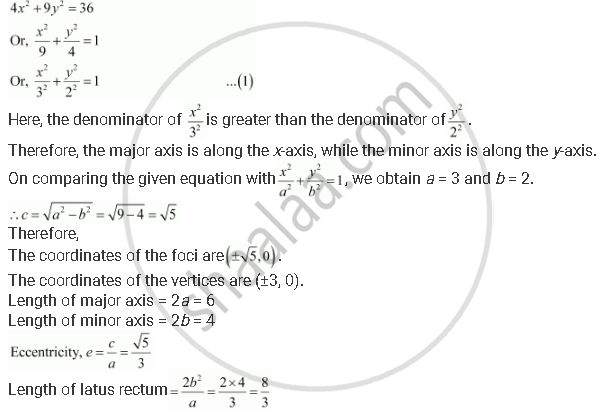CBSE (Arts) Class 11CBSE
Share
Notifications

View all notifications
Books Shortlist
Your shortlist is empty

# Find the Coordinates of the Foci, the Vertices, the Length of Major Axis, the Minor Axis, the Eccentricity and the Length of the Latus Rectum of the Ellipse 4x2 + 9y2 = 36 - CBSE (Arts) Class 11 - Mathematics

Login
Create free account

Forgot password?
ConceptEllipse Relationship Between Semi-major Axis, Semi-minor Axis and the Distance of the Focus from the Centre of the Ellipse

#### Question

Find the coordinates of the foci, the vertices, the length of major axis, the minor axis, the eccentricity and the length of the latus rectum of the ellipse 4x2 + 9y2 = 36

#### Solution

The given equation is 4x2 + 9y2 = 36.

It can be written asIs there an error in this question or solution?

#### APPEARS IN

Solution Find the Coordinates of the Foci, the Vertices, the Length of Major Axis, the Minor Axis, the Eccentricity and the Length of the Latus Rectum of the Ellipse 4x2 + 9y2 = 36 Concept: Ellipse - Relationship Between Semi-major Axis, Semi-minor Axis and the Distance of the Focus from the Centre of the Ellipse.
S